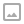☕ 10 分钟

# 自顶向下的设计

stateDiagram
watch: 观察
chase: 截球
kick: 回踢
[*] --> watch

watch --> chase: 球足够近
chase --> watch: 球跟丢了
chase --> kick: 球在跟前了
kick --> watch: 踢到了球或者球跟丢了


# 分而治之

• 全局：保持自己在场地内；确定球的位置；
• 观察：进入观察模式前需要返回出发点；
• 截球、踢球：小车需要向球移动。

## 向球移动

### 截球

• 球在场地上的位置并不容易获得，在场地上没有辅助标志的情况下，这需要同时知道球相对于车子的位置和车子在场地上的位置，计算比较复杂，误差累计较大；
• 球走得未必是直线，因为球会旋转，打滑，地面未必平整，同理，小车也未必一直走直线；

$$f(\Delta y) = K_p \Delta y$$

$$f(\Delta y) = K_p \Delta y + K_i \int_0^t \Delta y \cdot dt + K_d \frac{d}{dt} \Delta y$$

• $t$为时间；
• $K_p, K_i, K_d$分别为比例单元、积分单元、微分单元的系数；
• $\Delta y$是系统的目前误差；
• $\int_0^t \Delta y \cdot dt$代表了系统过去累计的误差；
• $\frac{d}{dt} \Delta y$代表了系统的未来误差。

1. 获取当前的$\Delta y$；
2. 计算$v_y = f(\Delta y)$，更新小车的横向速度为$v_y$；
3. 返回步骤1，重复。

## 球在哪里

### 球相对于机器人小车的位置

$$\frac{物体实际尺寸}{物体在相机传感器上的尺寸} = \frac{物体到相机的距离}{焦距}$$

$$L_{实际} = K_{相机} \cdot \frac{r_{实际}}{r_{图}}$$

$$\theta_{偏航} = 96 \cdot (\frac{x_{图}}{X_{图}} - 0.5)$$

\begin{aligned}\Delta x &= L_{实际} \cdot \cos\theta_{偏航}\\ \Delta y &= L_{实际} \cdot \sin\theta_{偏航} \end{aligned}

# 自顶向下的设计（再一次）

stateDiagram
watch: 观察
chase: 截球
kick: 回踢
[*] --> watch

watch --> chase: 球距离小车小于1.2m
chase --> watch: 前挡板检测到碰撞\n已经3秒没看到球\n球距离小车大于1.4m\n小车已经到达场地边界\n（满足任一条件即可）
chase --> kick: 球距离小车小于0.3m
kick --> watch: 前挡板检测到碰撞\n已经3秒没看到球\n球距离小车大于1.4m\n小车已经到达场地边界\n（满足任一条件即可）


# 参考资料nanmu42